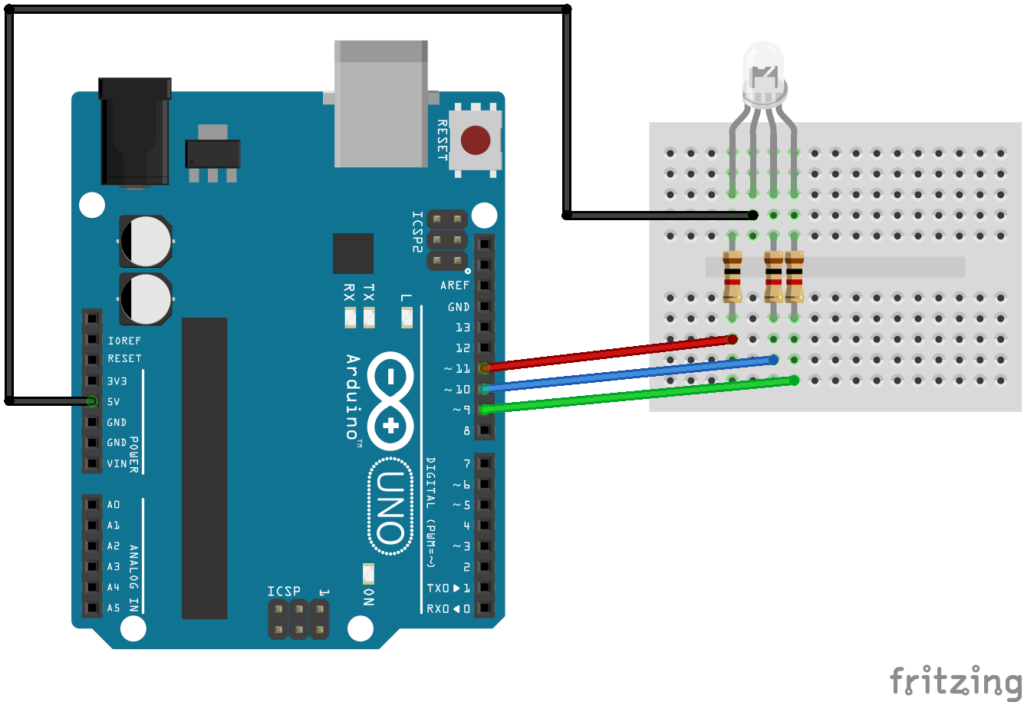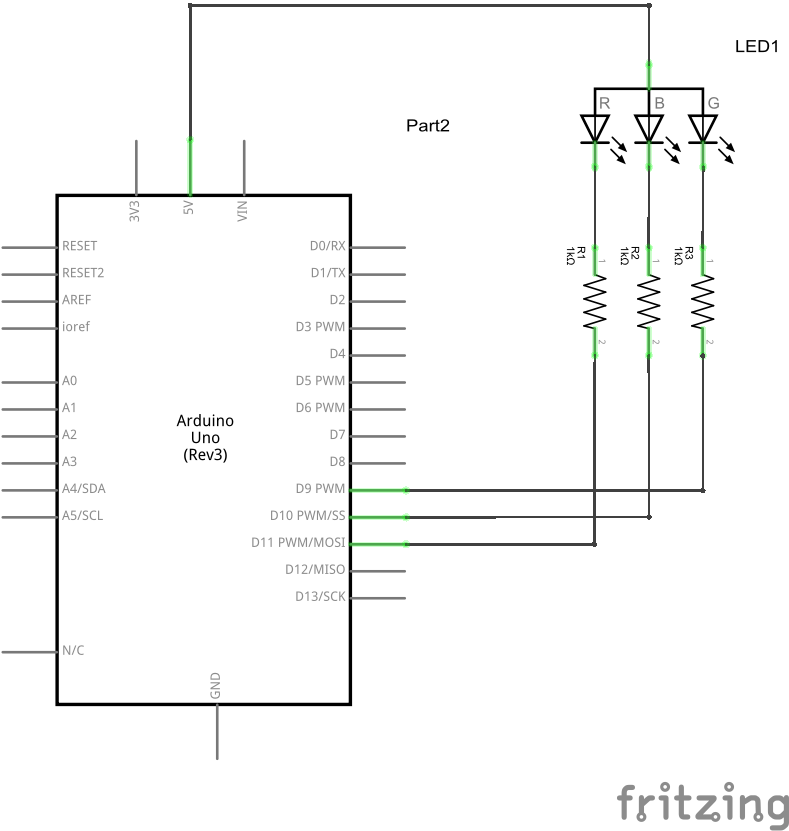Arduino for beginners : for loops

Arduino for beginners : for loops

The for statement is used to repeat a block of statements enclosed in curly braces. An increment counter is usually used to increment and terminate the loop. The for statement is useful for any repetitive operation, and is often used in combination with arrays to operate on collections of data/pins.
There are three parts to the for loop:

for (initialization; condition; increment)
{
//statement(s);
}

The initialization happens first and exactly once. Each time through the loop, the condition is tested; if it’s true, the statement block, and the increment is executed, then the condition is tested again. When the condition becomes false, the loop ends.

Code Examples

 Source code```void setup()
{
int i;
Serial.begin(9600);
for (i = 0; i < 10; i++)
{
Serial.print("i = ");
Serial.println(i);
}
}
void loop() {
}```

Open the serial monitor and you should see the following output

i = 0
i = 1
i = 2
i = 3
i = 4
i = 5
i = 6
i = 7
i = 8
i = 9

In these examples we will connect an RGB led to our Arduino, this will be used in several examples. Here is a breadboard layout and schematic, we used a common anode typeWe will only use the red led of the RGB led in these examples

 Source code```//change the brightness of an LED
int redled = 11;
void setup()
{
pinMode(redled, OUTPUT); //set the LED pin as an output
Serial.begin(9600);
for (int i=1; i <= 255; i++)
{
Serial.println("loop = ");
Serial.println(i);
analogWrite(redled, i);
delay(10);
}
}
void loop()
{
}```

As this is in the setup() it will only run once, changing this to the loop() will mean the led continuously runs through the for loop and fading the LED

 Source code```//change the brightness of an LED
int redled = 11;
void setup()
{
pinMode(redled, OUTPUT); //set the LED pin as an output
}
void loop()
{
for (int i=1; i <= 255; i++)
{
analogWrite(redled, i);
delay(10);
}
}```

In this example we will flash the red of an RGB led on and off 10 times

 Source code```//flash the red led of the RGB led 10 times
int redled = 11;

void setup()
{
Serial.begin(9600);
pinMode(redled, OUTPUT); //set the LED pin as an output
//this will run 10 times
for (int i=1; i <= 10; i++)
{
Serial.print("loop = ");
Serial.println(i);
digitalWrite(redled, LOW); // led on
delay(500);
digitalWrite(redled, HIGH); //led off
delay(500);
}
}

void loop()
{
}```